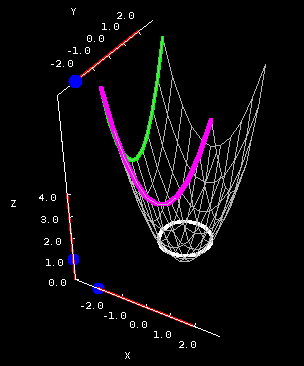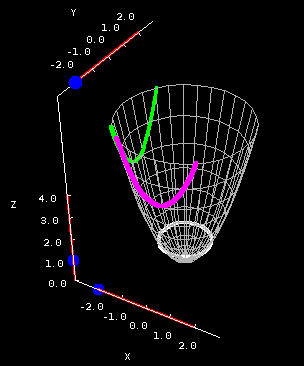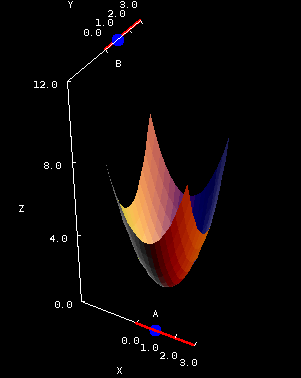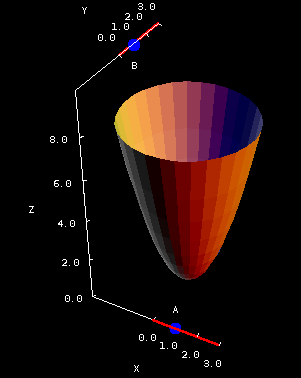# Math Insight

### The elliptic paraboloid

#### The elliptic paraboloid

Equation: $z=Ax^2+By^2$
(where A and B have the same sign)

This is probably the simplest of all the quadric surfaces, and it's often the first one shown in class. It has a distinctive “nose-cone” appearance.

This surface is called an elliptic paraboloid because the vertical cross sections are all parabolas, while the horizontal cross sections are ellipses. Occasionally we get sloppy and just refer to it simply as a paraboloid; that wouldn't be a problem, except that it leads to confusion with the hyperbolic paraboloid.

The cross sections below are for the simplest possible elliptic paraboloid: $$z = x^2 + y^2.$$Elliptic paraboloid cross sections. The elliptic paraboloid $z = x^2 + y^2$ is plotted on a square domain $-2 \le x \le 2, -2 \le y \le 2$ in the first panel and on the circular domain determined by $z \le 8$ in the second panel. You can drag the blue points on the sliders to change the location of the different types of cross sections.

One important feature of the vertical cross sections is that the parabolas all open in the same direction. That isn't true for hyperbolic paraboloids!

Note that in this case, the horizontal cross sections are actually circles, but this isn't always the case. In fact, whenever $A$ and $B$ are not equal, the paraboloid will be wider in one direction than the other.

You can use the below applet to investigate how these coefficients affect the shape of the surface. On the left, it shows the paraboloid $z = A x^2 + B y^2$ over the square domain \begin{gather*} -2 \le x \le 2\\ -2 \le y \le 2. \end{gather*} On the right, the domain is a disk, and it shows the portion of the paraboloid for which $0 \le z \le 8$. When you change A and B, the domain will change accordingly.Elliptic paraboloid coefficients. The paraboloid $z = A x^2 + B y^2$ is plotted over the square domain $-2 \le x \le 2, -2 \le y \le 2$ in the first panel. In the second panel, the same paraboloid is plotted over the elliptical domain for which $0 \le z \le 8$, a domain whose shape depends on the coefficients $A$ and $B$. You can drag the points to change $A$ and $B$, which are constrained to be positive.

Here are a few things to think about:

• What happens if either $A$ or $B$ is 0? What if they both are? Should any of these objects be called “elliptic” paraboloids?
• What would happen if the sliders included negative values for $A$ and $B$? (Click here to experiment and see if you're right.)

The elliptic paraboloid was used to motivate the notion of level curves. Can you see that the level curves are equivalent to the above horizontal cross sections?